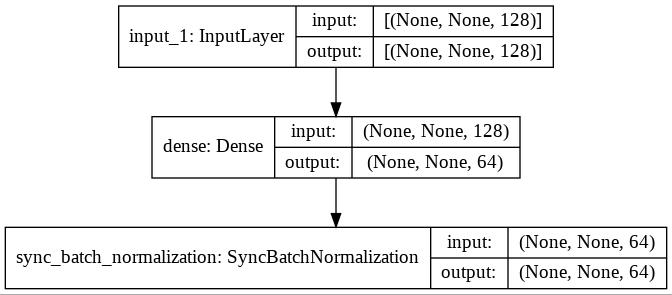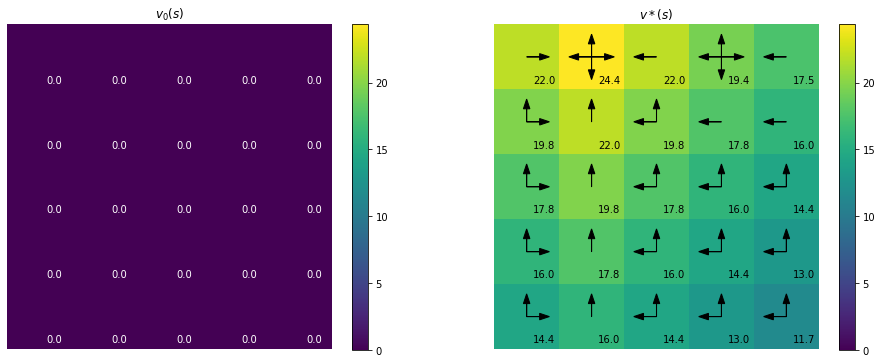### Speeding Up Diffusion Sampling

An important disadvantage with diffusion models is that a large number forward passes - typically 1000 or more - are needed to generate samples, making inference slow and expensive. In this post we will look at a couple of different methods that have been developed to speed up inference.### An Introduction to Diffusion

In this blog we will learn about the basic diffusion-based generative model introduced in Denoising Diffusion Probabilistic Models (DDPM). This post will cover the basics at a relatively high level but along the way there will be optional exercises that will help you to delve deeper into the mathematical details.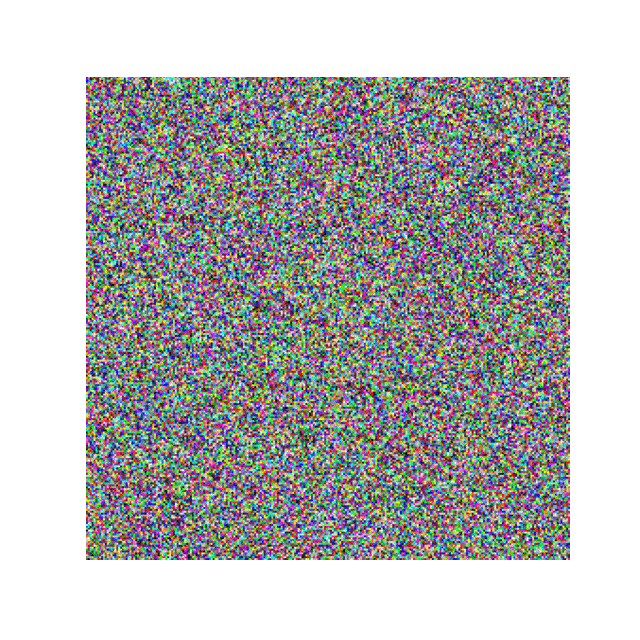### Veg2vec - word embeddings for plant-based foods with health benefits

One of the most exciting uses of machine learning is scientific discovery. Sometimes long before a discovery is officially made people have written about similar ideas and one could speculate what might have happened if some dots had been connected sooner. This is exactly what the mat2vec model (code, paper) seeks to do with the help of AI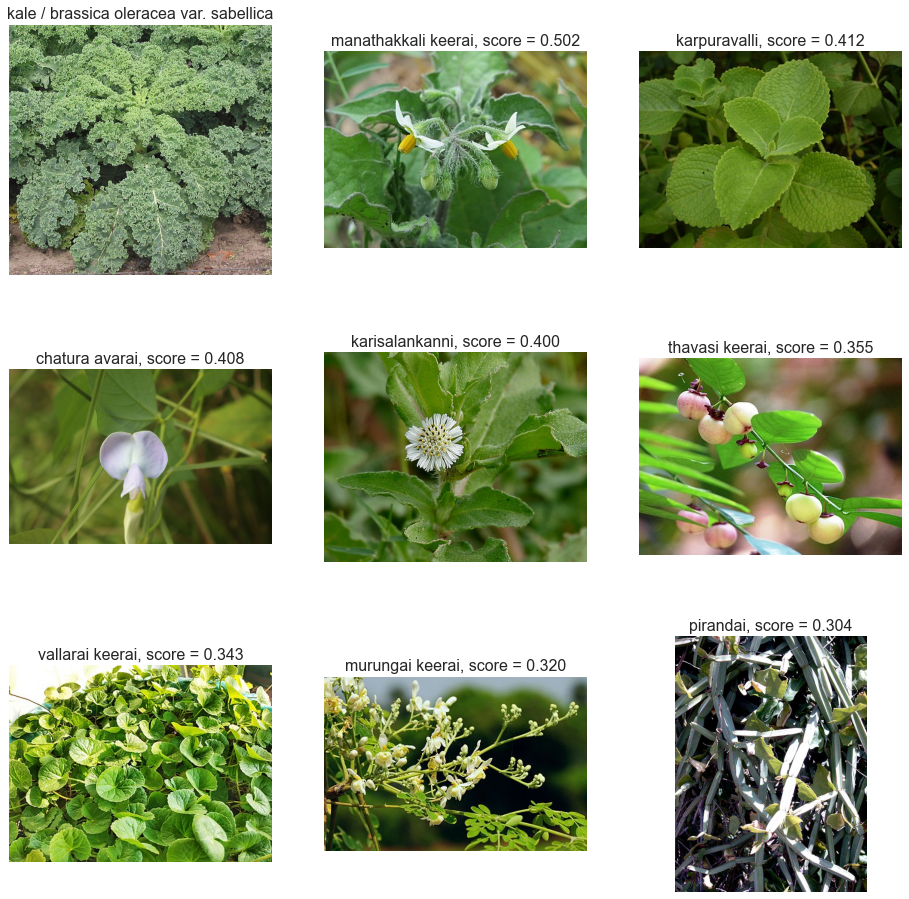### A quick overview of GLIDE

You have probably come across DALL-E 2 a large-scale image generation model from OpenAI. If not, you can read about it in their blog. DALL-E 2 uses a class of models known as diffusion models. The DALL-E 2 paper does not go into a lot of detail about the model architecture since it mostly extends an earlier architecture GLIDE. This article provides a brief introduction to the GLIDE architecture.### REINFORCE on the short-corridor gridworld, Part 1 (RL S&B Chapter 13)

In this tutorial we will demonstrate how to implement Example 13.1 of Reinforcement Learning (Sutton and Barto).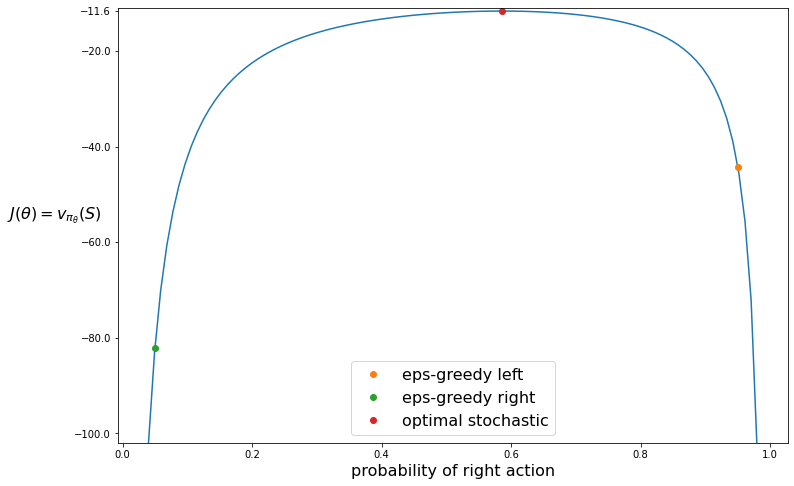### Spherical Interpolation

$\newcommand{\vv}{\mathbf{v}}$ In machine learning applications you sometimes want to interpolate vectors in a normalised latent space such as when interpolating between two images in a generative model. An appropriate method for doing this is spherical interpolation. In this post we will derive the formula for this method and show how it differs from linear interpolation.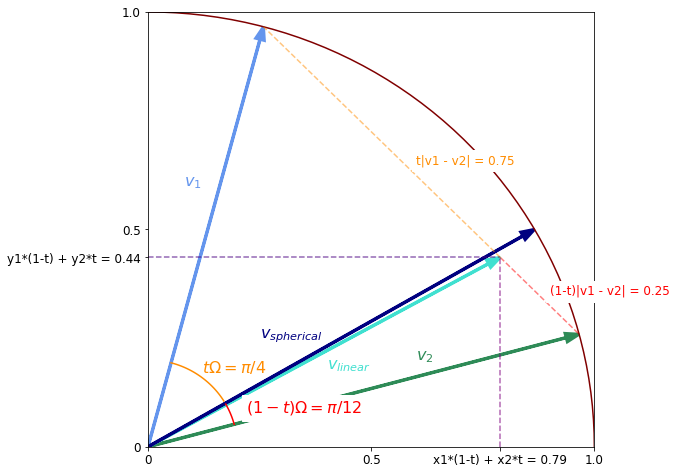### A Quick Introduction to Importance Sampling

You might have encountered the term importance sampling in a machine learning paper. This post provides a quick hands-on introduction to this topic. Hopefully after reading it you will have know understand how to use this technique in practice.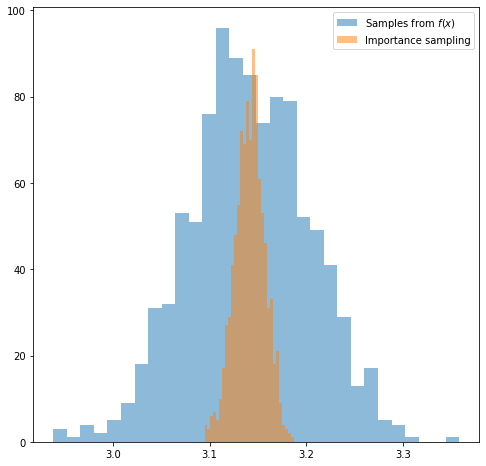### Gradient Monte Carlo for Value Function Approximation (RL S&B Example 9.1)

In this post we will implement Example 9.1 from Chapter 9 Reinforcement Learning (Sutton and Barto). This is an example of on-policy prediction with approximation which means that you try to approximate the value function for the policy you are following right now. We will be using Gradient Monte Carlo for approximating $v_\pi(s)$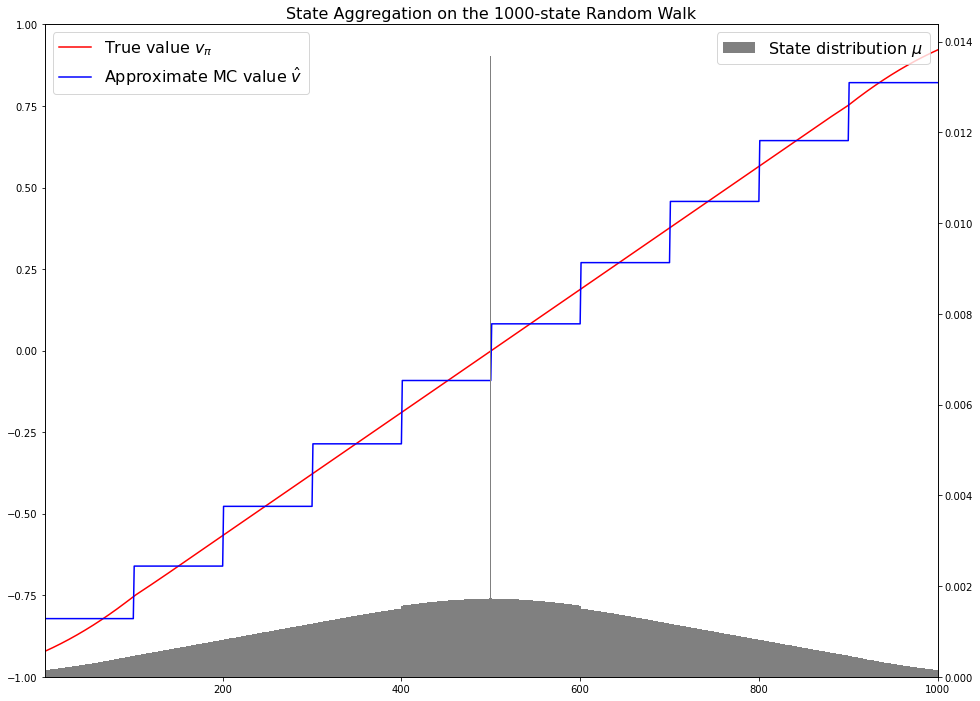### Distributed BatchNorm in TensorFlow

In this post we will see an example of how BatchNorm works when running distributed training with TensorFlow using TPUs and tf.keras.layers. This post assumes you know BatchNormalisation works.